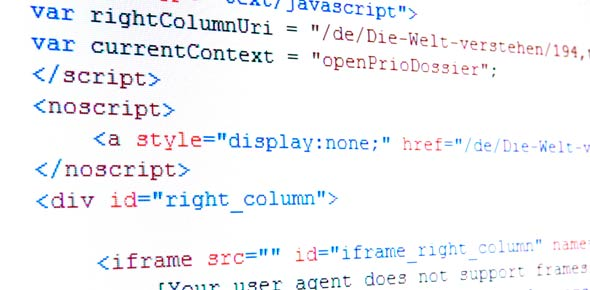# Computer Science Quiz: Programming Questions

9 Questions | Total Attempts: 49Settings• 1.
Consider the following method:public static boolean mystery(int [] a) {     for (int i = 0; i < a.length - 1; i += 1) {          if a[i] > a[i + 1) {                return false;          }     }     return true;}If I create the following arrayint [] foo = {0, 4, 7, 18, 34, 50, 51, 95, 94}What is returned by mystery(foo);
• A.

True

• B.

False

• C.

Nothing, the code has a syntax error

• D.

Nothing, the code will cause an exception

• 2.
Assume the following classpublic class Foobar {     private int x = 6;     private final y = 8;     public int z = -1;     public int getX() { return x; }     public int getXZ() { return z + z;}}Which of the following are syntactically correct?
• A.

Null and void

• B.

And void

• C.

And

• D.

Compile error

• E.

Run-time exception

• 3.
What is printed by the following code?int x = -8, y = 4, z = 13;char a = 'a', d = 'D';and examine the following conditions:if  (a != d || x != -8) {     System.out.println(z / 4);} else {     System.out.println(z % 4);}
• A.

0.25

• B.

1

• C.

3.25

• D.

3

• 4.
Assume a and b are integer variables that have been assign values. The expression: !((a < b) && (b < 7)) Is equivalent to which of the following
• A.

(a >= b) || (b >= 7)

• B.

(a < b) || (b < 7)

• C.

(a >= b) || (b < 7)

• D.

(a >= b) && (b >= 7)

• E.

(a > b) && (b > 7)

• 5.
Consider the following method:public String stringer(String s) {      String d = "";      for int (i = 0; i < s.length(); i++)             if (s.charAt(i) != 'k') {                  d = d + s.charAt(i);             } else {                  d = d + "ck";             }       }       return d;}What is returned by the following call: stringer("Krazy kat");
• A.

Krazy kay

• B.

Ckrazy ckat

• C.

Krazy ckat

• D.

Krazy Kat

• E.

Ckrazy ckat

• 6.
What does the following method do?public String jake(int [] a) {    int [] r = new r[2*a.length];    int m = 0; n = a.length;    while (m <= a.length - 1)  {         r = a[m];         r[n] = a[m];         m++;         n--;    }    return r;}
• A.

Returns a copy of the original array in reverse order

• B.

Returns a copy of the original array that has twice as many elements

• C.

Returns a new array that's a concatenation of the original array appended on itself

• D.

Returns a new array that's a concatenation of the original array and it's mirror image

• 7.
Consider the following code: String usa = "Uncle Sam"; String uk = "The Queen"; String mystery = usa.substring(uk.indexOf("Q")); What is the value of mystery?
• 8.
Consider the following code:  Random r as new Random() int d1 = r.nextInt(5) + 1; int d2 = r.nextInt(5) + 1; if (d1 != d2) {     if (d1 + d1 == 7) {         System.out.println("Craps!!");     } else if (d1 + d2 < 7) {         System.out.println("Advance to Go!!");     } else {         System.out.println("Bingo!!");     } } else {     System.out.println("Go to Jail!!"); } If d1 is randomly assigned a 5 and d2 is randomly assigned a 1, what does the code print out?
• 9.
Assume the following classpublic class Foobar {     private int x = 6;     public final y = 8;     private int z = -1;     public int    getX() { return x; }     public int    getXZ() { return z + z;}     public void setZ(int pz) { z = pz;} }Assuming we create the following object:Foobar f = new Foobar();Which of the following are syntactically correct? (Check all that apply)
• A.

F.y = 23

• B.

F.setZ(0);

• C.

Int a = f.x;

• D.

Int a = f.y + * f.getX();

Related TopicsBack to top